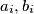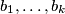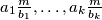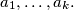### IMO Shortlist 1974 problem 7

Kvaliteta:
Avg: 0,0
Težina:
Avg: 0,0
Let$a_i, b_i$ be coprime positive integers for$i = 1, 2, \ldots , k$, and$m$ the least common multiple of$b_1, \ldots , b_k$. Prove that the greatest common divisor of$a_1 \frac{m}{b_1} , \ldots, a_k \frac{m}{b_k}$ equals the greatest common divisor of$a_1, \ldots , a_k.$
Izvor: Međunarodna matematička olimpijada, shortlist 1974